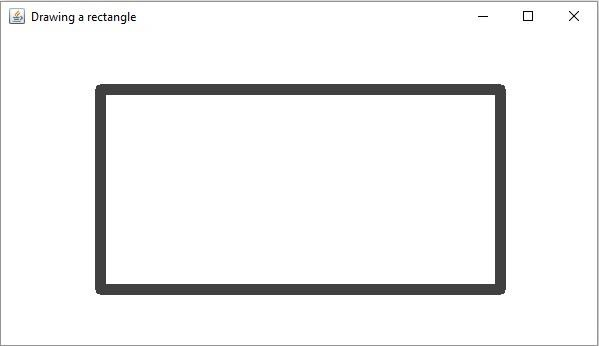# How to draw a rectangle in OpenCV using Java?

The org.opencv.imgproc package of Java OpenCV library contains a class named Imgproc. To draw a rectangle you need to invoke the rectangle() method of this class. This method accepts the following parameters −

• A Mat object representing the image on which the rectangle is to be drawn.

• Two Point objects representing the vertices of the rectangle that is to be drawn.

• A Scalar object representing the color of the rectangle(BGR).

• An integer representing the thickness of the rectangle(default:1).

## Example

import org.opencv.core.Core;
import org.opencv.core.Mat;
import org.opencv.core.Point;
import org.opencv.core.Scalar;
import org.opencv.highgui.HighGui;
import org.opencv.imgcodecs.Imgcodecs;
import org.opencv.imgproc.Imgproc;
public class DrawingRectangle {
public static void main(String args[]) {
//Reading the source image in to a Mat object
//Drawing a Rectangle
Point point1 = new Point(100, 100);
Point point2 = new Point(500, 300);
Scalar color = new Scalar(64, 64, 64);
int thickness = 10;
Imgproc.rectangle (src, point1, point2, color, thickness);
//Saving and displaying the image
Imgcodecs.imwrite("arrowed_line.jpg", src);
HighGui.imshow("Drawing a rectangle", src);
HighGui.waitKey();
}
}

## Output

On executing, the above program generates the following window −